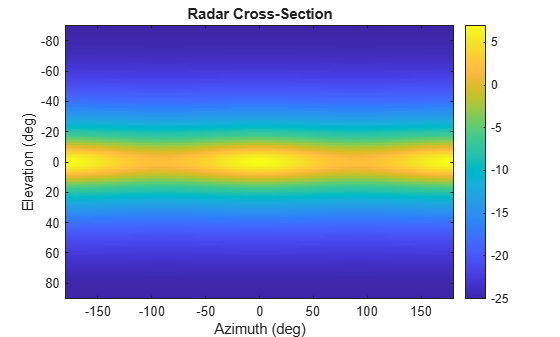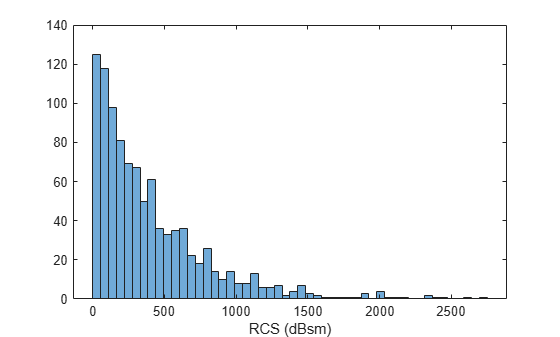# rcsSignature

Since R2021a

## Description

`rcsSignature` creates a radar cross-section (RCS) signature object. You can use this object to model an angle-dependent and frequency-dependent radar cross-section pattern. The radar cross-section determines the intensity of reflected radar signal power from a target. The object models both polarized and non-polarized signals. The object support several Swerling fluctuation models.

## Creation

### Syntax

``rcssig = rcsSignature``
``rcssig = rcsSignature(Name,Value)``

### Description

``` `rcssig = rcsSignature` creates an `rcsSignature` object with default property values.```

example

````rcssig = rcsSignature(Name,Value)` sets object properties using one or more `Name,Value` pair arguments. `Name` is a property name and `Value` is the corresponding value. `Name` must appear inside single quotes (`''`). You can specify several name-value pair arguments in any order as `Name1,Value1,...,NameN,ValueN`. Any unspecified properties take default values.```

Note

You can only set property values of `rcsSignature` when constructing the object. The property values are not changeable after construction.

## Properties

expand all

To enable polarization, set this property to `true` to enable polarization. Set this property to `false` to ignore polarization.

Data Types: `logical`

Polarization scattering matrix form, specified as `'Full'` or `'Symmetric'`.

• `'Full'` makes no assumptions about the cross-polarization terms and the polarization matrix components must all be specified (`ShhPattern`, `SvvPattern`, `ShvPattern`, `SvhPattern`). When this input method is selected, the `ShvPattern` and `SvhPattern` properties are applicable.

• `'Symmetric'` assumes reciprocity (monostatic geometry) and that the cross-polarization terms are equal (`ShvPattern` = `SvhPattern`). When this input method is selected, the `ShvPattern` property is available. The `SvhPattern` property is not available and is assumed to be equal to the `ShvPattern` property.

#### Dependencies

To enable this property, set the `EnablePolarization` property to `true`.

Sampled radar cross-section (RCS) pattern, specified as a scalar, a Q-by-P real-valued matrix, or a Q-by-P-by-K real-valued array. The pattern is an array of RCS values defined on a grid of elevation angles, azimuth angles, and frequencies. Azimuth and elevation are defined in the body frame of the target.

• Q is the number of RCS samples in elevation.

• P is the number of RCS samples in azimuth.

• K is the number of RCS samples in frequency.

Q, P, and K usually match the length of the vectors defined in the `Elevation`, `Azimuth`, and `Frequency` properties, respectively, with these exceptions:

• To model an RCS pattern for an elevation cut (constant azimuth), you can specify the RCS pattern as a Q-by-1 vector or a 1-by-Q-by-K matrix. Then, the elevation vector specified in the `Elevation` property must have length 2.

• To model an RCS pattern for an azimuth cut (constant elevation), you can specify the RCS pattern as a 1-by-P vector or a 1-by-P-by-K matrix. Then, the azimuth vector specified in the `Azimuth` property must have length 2.

• To model an RCS pattern for one frequency, you can specify the RCS pattern as a Q-by-P matrix. Then, the frequency vector specified in the `Frequency` property must have length-2.

Example: `[10,0;0,-5]`

Data Types: `double`

Polarization scattering matrix co-polarized HH component, where HH represents horizontal transmission and horizontal reception. Q is the length of the vector in the `Elevation` property. P is the length of the vector in the `Azimuth` property. If the pattern is three-dimensional, then K is the length of the vector in the `Frequency` property.

When defining patterns for azimuth or elevation only cuts, P or Q can equal 1, but the corresponding `Azimuth` or `Elevation` property must still be a 2-element vector. In this case, the `Azimuth` or `Elevation` property defines the view angles over which the pattern is considered valid for the pattern's dimension with length 1.

#### Dependencies

To enable this property, set the `EnablePolarization` property to `true`.

Data Types: `double`
Complex Number Support: Yes

Polarization scattering matrix co-polarized VV component, where VV represents vertical transmission and vertical reception. Q is the length of the vector in the `Elevation` property. P is the length of the vector in the `Azimuth` property. If the pattern is three-dimensional, then K is the length of the vector in the `Frequency` property.

When defining patterns for azimuth or elevation only cuts, P or Q can equal 1, but the corresponding `Azimuth` or `Elevation` property must still be a 2-element vector. In this case, the `Azimuth` or `Elevation` property defines the view angles over which the pattern is considered valid for the pattern's dimension with length 1.

#### Dependencies

To enable this property, set the `EnablePolarization` property to `true`.

Data Types: `double`
Complex Number Support: Yes

Polarization scattering matrix co-polarized HV component, where HV represents horizontal transmission and vertical reception. Q is the length of the vector in the `Elevation` property. P is the length of the vector in the `Azimuth` property. If the pattern is three-dimensional, then K is the length of the vector in the `Frequency` property.

When defining patterns for azimuth or elevation only cuts, P or Q can equal 1, but the corresponding `Azimuth` or `Elevation` property must still be a 2-element vector. In this case, the `Azimuth` or `Elevation` property defines the view angles over which the pattern is considered valid for the pattern's dimension with length 1.

#### Dependencies

To enable this property, set the `EnablePolarization` property to `true`.

Data Types: `double`
Complex Number Support: Yes

Polarization scattering matrix co-polarized HV component, where VH represents vertical transmission and horizontal reception. Q is the length of the vector in the `Elevation` property. P is the length of the vector in the `Azimuth` property. If the pattern is three-dimensional, then K is the length of the vector in the `Frequency` property.

When defining patterns for azimuth or elevation only cuts, P or Q can equal 1, but the corresponding `Azimuth` or `Elevation` property must still be a 2-element vector. In this case, the `Azimuth` or `Elevation` property defines the view angles over which the pattern is considered valid for the pattern's dimension with length 1.

#### Dependencies

To enable this property, set the `EnablePolarization` property to `true` and the `CrossPolarization` property to `'Full'`.

Data Types: `double`
Complex Number Support: Yes

Azimuth angles used to define the angular coordinates of each column of the matrix or array, specified by the `Pattern` property. Specify the azimuth angles as a length-P vector. P must be greater than two. Angle units are in degrees.

When the `Pattern` property defines an elevation cut, `Azimuth` must be a 2-element vector defining the minimum and maximum azimuth view angles over which the elevation cut is considered valid.

Example: `[-45:0.5:45]`

Data Types: `double`

Elevation angles used to define the coordinates of each row of the matrix or array, specified by the `Pattern` property. Specify the elevation angles as a length-Q vector. Q must be greater than two. Angle units are in degrees.

When the `Pattern` property defines an azimuth cut, `Elevation` must be a 2-element vector defining the minimum and maximum elevation view angles over which the azimuth cut is considered valid.

Example: `[-30:0.5:30]`

Data Types: `double`

Frequencies used to define the applicable RCS for each page of the `Pattern` property, specified as a K-element vector of positive scalars. K is the number of RCS samples in frequency. K must be no less than two. Frequency units are in hertz.

When the `Pattern` property is a matrix, `Frequency` must be a 2-element vector defining the minimum and maximum frequencies over which the pattern values are considered valid.

Example: `[0:0.1:30]`

Data Types: `double`

Fluctuation models, specified as `'Swerling0'`, `'Swerling1'` or `'Swerling3'`. Swerling cases 2 and 4 are not modeled as these are determined how the target is sample, not an inherent target property.

ModelDescription
`'Swerling0'`The target RCS is assumed to be non-fluctuating. In this case the instantaneous RCS signature value retrieved by the value method is deterministic. This model represents ideal radar targets with an RCS that remains constant in time across the range of aspect angles of interest, e.g., a conducting sphere and various corner reflectors.
`'Swerling1'`The target is assumed to be made up of many independent scatterers of equal size. This model is typically used to represent aircraft. The instantaneous RCS signature value returned by the value method in this case is a random variable distributed according to the exponential distribution with a mean determined by the `Pattern` property.
`'Swerling3'`The target is assumed to have one large dominant scatterer and several small scatterers. The RCS of the dominant scatterer equals 1+sqrt(2) times the sum of the RCS of other scatterers. This model can be used to represent helicopters and propeller driven aircraft. In this case the instantaneous RCS signature's value returned by the value method is a random variable distributed according to the 4th degree chi-square distribution with mean determined by the `Pattern` property.

Data Types: `char` | `string`

## Object Functions

 `value` Radar cross-section at specified angle and frequency `toStruct` Convert to structure

## Examples

collapse all

Specify the radar cross-section (RCS) of a triaxial ellipsoid and plot RCS values along an azimuth cut.

Specify the lengths of the axes of the ellipsoid. Units are in meters.

```a = 0.15; b = 0.20; c = 0.95;```

Create an RCS array. Specify the range of azimuth and elevation angles over which RCS is defined. Then, use an analytical model to compute the radar cross-section of the ellipsoid. Create an image of the RCS.

```az = [-180:1:180]; el = [-90:1:90]; rcs = rcs_ellipsoid(a,b,c,az,el); rcsdb = 10*log10(rcs); imagesc(az,el,rcsdb) title('Radar Cross-Section') xlabel('Azimuth (deg)') ylabel('Elevation (deg)') colorbar```Create an `rcsSignature` object and plot an elevation cut at $3{0}^{\circ }$ azimuth.

```rcssig = rcsSignature('Pattern',rcsdb,'Azimuth',az,'Elevation',el,'Frequency',[300e6 300e6]); rcsdb1 = value(rcssig,30,el,300e6); plot(el,rcsdb1) grid title('Elevation Profile of Radar Cross-Section') xlabel('Elevation (deg)') ylabel('RCS (dBsm)')``````function rcs = rcs_ellipsoid(a,b,c,az,el) sinaz = sind(az); cosaz = cosd(az); sintheta = sind(90 - el); costheta = cosd(90 - el); denom = (a^2*(sintheta'.^2)*cosaz.^2 + b^2*(sintheta'.^2)*sinaz.^2 + c^2*(costheta'.^2)*ones(size(cosaz))).^2; rcs = (pi*a^2*b^2*c^2)./denom; end```

Import the radar cross-section (RCS) measurements of a 1/5th scale Boeing 737. Load the RCS data into an `rcsSignature` object. Assume the RCS follows a Swerling 1 distribution.

```load('RCSSignatureExampleData.mat','boeing737'); rcs = rcsSignature('Pattern',boeing737.RCSdBsm, ... 'Azimuth', boeing737.Azimuth,'Elevation',boeing737.Elevation, ... 'Frequency',boeing737.Frequency,'FluctuationModel','Swerling1');```

Set the seed of the random number generator for reproducibility of example.

`rng(3231)`

Plot sample RCS versus azimuth angle.

```plot(rcs.Azimuth,rcs.Pattern) xlabel('Azimuth (deg)'); ylabel('RCS (dBsm)') title('Measured RCS from 1/5th scale Boeing 737 model')```Construct an RCS histogram and display the mean value.

```N = 1000; val = zeros(1,N); for k = 1:N [val(k),expval] = value(rcs,-5,0,800.0e6); end```

Convert to power units.

`mean(db2pow(val))`
```ans = 406.9799 ```
```histogram(db2pow(val),50) xlabel("RCS (dBsm)")```Richards, Mark A. Fundamentals of Radar Signal Processing. New York, McGraw-Hill, 2005.